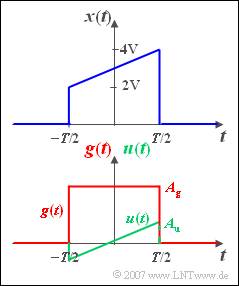# Exercise 3.6: Even and Odd Time Signals"Wedge function" as well as an even and an odd time signal

We are looking for the spectrum  $X(f)$  of the pulse  $x(t)$ sketched opposite, which rises linearly from  $2\hspace{0.05cm} \text{V}$  to  $4\hspace{0.05cm} \text{V}$  in the range from  $–T/2$  to  $+T/2$  and is zero outside.

The spectral functions of the signals  $g(t)$  and  $u(t)$  shown below are assumed to be known:

• The even (German:  gerade) rectangular time function  $g(t)$  has the spectrum
$$G( f ) = A_g \cdot T \cdot {\mathop{\rm si}\nolimits}( { {\rm{\pi }}fT} ) \hspace{0.3cm} {\rm{with}}\hspace{0.3cm} {\mathop{\rm si}\nolimits}( x ) = {\sin ( x )}/{x}.$$
• The spectrum of the odd (German:  ungerade) function  $u(t)$  is:
$$U( f ) = - {\rm{j}} \cdot \frac{ {A_u \cdot T}}{ {2{\rm{\pi }}fT}}\big[ {{\mathop{\rm si}\nolimits} ( { {\rm{\pi }}fT} ) - \cos ( { {\rm{\pi }}fT} )} \big].$$

Hints:

### Questions

1

Calculate the (imaginary) spectral values of the odd signal  $u(t)$  at the frequencies  $f = 0.5\,\text{kHz}$  and  $f = 1\,\text{kHz}$.

 ${\rm Im}\big[U(f=0.5 \,\text{kHz})\big] \ = \$  $\text{mV/Hz}$ ${\rm Im}\big[U(f=1.0 \,\text{kHz})\big]\ = \$  $\text{mV/Hz}$

2

What is the spectral value of  $u(t)$  at the frequency  $f = 0$?     Hint: Think before you calculate.

 ${\rm Im}\big[U(f=0)\big]\ = \$  $\text{mV/Hz}$

3

Using the result from  (1)  calculate the spectral value of the signal  $x(t)$  at the frequency  $f=0.5 \,\text{kHz}$.

 ${\rm Re}\big[X(f=0.5 \,\text{kHz})\big]\ = \$  $\text{mV/Hz}$ ${\rm Im}\big[X(f=0.5 \,\text{kHz})\big]\ = \$  $\text{mV/Hz}$

### Solution

#### Solution

(1)  For  $f \cdot T = 0.5$  one obtains from the given equation:

$$U( {f = 0.5\;{\rm{kHz}}} ) = - {\rm{j}} \cdot \frac{ {A_u \cdot T}}{ {\rm{\pi }}} \cdot {\mathop{\rm si}\nolimits} ( {{ {\rm{\pi }}}/{2}} ) = - {\rm{j}} \cdot \frac{2}{ { {\rm{\pi }}^{\rm{2}} }} \cdot A_{\rm u} \cdot T.$$
• The imaginary part is  ${\rm Im}[U(f=0.5 \,\text{kHz})]\; \underline{\approx 0.2 \,\text{mV/Hz}}$.
• In contrast, the  $\rm si$–function at  $f \cdot T = 1$  yields the value zero, while the cosine is equal to  $-1$.  Thus with  $A_u = 1\,\text{V}$  and  $T = 1\,\text{ms}$  one obtains:
$$U( {f = 1\;{\rm{kHz}}} ) = {\rm{j}} \cdot \frac{ {A_{\rm u} \cdot T}}{ { {\rm{2\pi }}}} \hspace{0.3 cm} \Rightarrow \hspace{0.3 cm} {\rm Re} [\text{...}] \hspace{0.15 cm}\underline{ = 0}, \hspace{0.3 cm}{\rm Im} [\text{...}] \hspace{0.15 cm}\underline{\approx 0.159 \;{\rm{mV/Hz}}}.$$

(2)  According to the  "Assignment Theorem", an odd time function  $u(t)$  always has an imaginary and at the same time odd spectrum  $U( { - f} ) = - U( f )$.  With the boundary transition  $f \rightarrow \infty$  follows from the given equation

$$U( f ) = - {\rm{j}} \cdot \frac{ {A_u \cdot T}}{ {2{\rm{\pi }}fT}}\big[ { {\mathop{\rm si}\nolimits} ( {{\rm{\pi }}fT} ) - \cos ( { {\rm{\pi }}fT} )} \big]$$

the result  $U(f = 0) = 0$.  Formally, one could confirm this result by applying l'Hospital's rule.

We proceed a little more pragmatically.

• For example, if we set  $f \cdot T = 0.01$, we obtain:

$$U( {f \cdot T = 0.01}) = -{\rm{j}} \cdot \frac{ {A_{\rm u} \cdot T}}{{0.02{\rm{\pi }}}}\big[ {{\mathop{\rm si}\nolimits} ( {0.01{\rm{\pi }}} ) - \cos ( {0.01{\rm{\pi }}} )} \big ] = - {\rm{j}} \cdot \frac{ {A{\rm u} \cdot T}}{{0.02{\rm{\pi }}}}( {0.999836 - 0.999507} ) \approx - {\rm{j}} \cdot 5 \cdot 10^{ - 6} \;{\rm{V/Hz}}{\rm{.}}$$

• For even smaller frequency values, the result also becomes smaller and smaller.
• You get to the result  $U(f = 0)\;\underline{ = 0}$  more quickly if you take into account that the integral over  $u(t)$  disappears.
• So you don't have to calculate at all.

(3)  The signal  $x(t)$  can be divided into an even and an odd part, which lead to the even real part and the odd imaginary part of  $X(f)$ :

• The even part is equal to the function  $g(t)$  with  $A_g = 3\,\text{V}$.  From this follows for the real part of the spectral value at  $f \cdot T = 0.5$:
$${\mathop{\rm Re}\nolimits} \left[ {X( {f \cdot T = 0.5} )} \right] = A_{\rm g} \cdot T \cdot {\mathop{\rm si}\nolimits} ( {{ {\rm{\pi }}}/{2}} ) \hspace{0.15 cm}\underline{= 1.91 \;{\rm{mV/Hz}}}{\rm{.}}$$
• The imaginary part results from the spectral function  $U(f)$  with $A_u = 1\,\text{V}$.  This was already calculated in subtask  (1):
$${\mathop{\rm Im}\nolimits} \left[ {X( {f \cdot T = 0.5} )} \right] \hspace{0.15 cm}\underline{\approx - 0.2 \;{\rm{mV/Hz}}}{\rm{.}}$$